# WA Inverse Discrete Wavelet Transform VI

LabVIEW 2014 Advanced Signal Processing Toolkit Help

Edition Date: June 2014

Part Number: 372656C-01

»View Product InfoDownload Help (Windows Only)

Owning Palette: Discrete Wavelet VIs

Computes the multi-level inverse discrete wavelet transform (DWT) and returns the reconstructed signal from the approximation coefficients and the detail coefficients. An interpolator with a factor 2 and the lowpass synthesis filters and the highpass synthesis filters implement the inverse DWT at each level. Wire data to the DWT coef input to determine the polymorphic instance to use or manually select the instance.

Examples

Use the pull-down menu to select an instance of this VI.

 Select an instance WA Inverse 1D Discrete Wavelet Transform (Waveform)WA Inverse 1D Discrete Wavelet Transform (Array)WA Inverse 2D Discrete Wavelet Transform

## WA Inverse 1D Discrete Wavelet Transform (Waveform)DWT coef specifies the approximation coefficients and the detail coefficients for the multi-level inverse discrete wavelet transform (DWT). You must organize the coefficients into a waveform starting with the approximation coefficients at the largest level followed by the detail coefficients at all levels in descending order.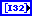length specifies the length of the approximation coefficients, the detail coefficients, and the signal. You must organize length into a 1D array starting with the length of the approximation coefficients at the largest level, the length of the detail coefficients at all levels in descending order, and the length of the signal.wavelet specifies the wavelet type of the discrete wavelet analysis. The default is db02. wavelet must be the same type as the wavelet type you selected in the WA Discrete Wavelet Transform VI to compute DWT coef. If you used the analysis filters input of the WA Discrete Wavelet Transform VI to specify wavelet, ignore this input and use the synthesis filters input of this VI to specify the synthesis filters of the wavelet.error in describes error conditions that occur before this node runs. This input provides standard error in functionality.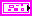synthesis filters specifies the coefficients of the lowpass synthesis filters and the highpass synthesis filters for the wavelet you specify. If you specify a value for synthesis filters, this VI ignores the settings in the wavelet input. You can use the Wavelet Design Express VI to design the analysis filters and the corresponding synthesis filters.lowpass specifies the coefficients of the lowpass synthesis filter, which this VI uses to filter the interpolated approximation coefficients in the wavelet reconstruction.highpass specifies the coefficients of the highpass synthesis filter, which this VI uses to filter the interpolated detail coefficients in the wavelet reconstruction.signal returns the reconstructed signal.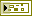error out contains error information. This output provides standard error out functionality.

## WA Inverse 1D Discrete Wavelet Transform (Array)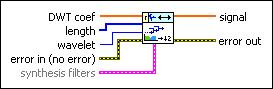DWT coef specifies the approximation coefficients and the detail coefficients for the multi-level inverse discrete wavelet decomposition (DWT). You must organize the coefficients into a 1D real array starting with the approximation coefficients at the largest level followed by the detail coefficients at all levels in descending order.length specifies the length of the approximation coefficients, the detail coefficients, and the signal. You must organize length into a 1D array starting with the length of the approximation coefficients at the largest level, the length of the detail coefficients at all levels in descending order, and the length of the signal.wavelet specifies the wavelet type of the discrete wavelet analysis. The default is db02. wavelet must be the same type as the wavelet type you selected in the WA Discrete Wavelet Transform VI to compute DWT coef. If you used the analysis filters input of the WA Discrete Wavelet Transform VI to specify wavelet, ignore this input and use the synthesis filters input of this VI to specify the synthesis filters of the wavelet.error in describes error conditions that occur before this node runs. This input provides standard error in functionality.synthesis filters specifies the coefficients of the lowpass synthesis filters and the highpass synthesis filters for the wavelet you specify. If you specify a value for synthesis filters, this VI ignores the settings in the wavelet input. You can use the Wavelet Design Express VI to design the analysis filters and the corresponding synthesis filters.lowpass specifies the coefficients of the lowpass synthesis filter, which this VI uses to filter the interpolated approximation coefficients in the wavelet reconstruction.highpass specifies the coefficients of the highpass synthesis filter, which this VI uses to filter the interpolated detail coefficients in the wavelet reconstruction.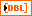signal returns the reconstructed signal.error out contains error information. This output provides standard error out functionality.

## WA Inverse 2D Discrete Wavelet Transform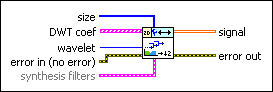size specifies the size of the reconstructed 2D signal as the number of rows followed by the number of columns.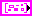DWT coef specifies the approximation coefficients and the detail coefficients for the multi-level inverse discrete wavelet transform. Each element of the array contains the 2D DWT results of one level. The ith element stores the approximation coefficients and the detail coefficients at level i+1.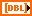low_low specifies the approximation coefficients from the lowpass analysis filtering on each row and each column. The low_low coefficients are a low-resolution approximation of the original 2D signal.low_high specifies the detail coefficients from the lowpass analysis filtering on each row and the highpass analysis filtering on each column. The high-frequency signal along the column direction influences the low_high coefficients.high_low specifies the detail coefficients from the highpass analysis filtering on each row and the lowpass analysis filtering on each column. The high-frequency signal along the row direction influences the high_low coefficients.high_high specifies the detail coefficients from the highpass analysis filtering on each row and each column. The high-frequency signal along the diagonal direction influences the high_high coefficients.wavelet specifies the wavelet type of the discrete wavelet analysis. The default is db02. wavelet must be the same type as the wavelet type you selected in the WA Discrete Wavelet Transform VI to compute DWT coef. If you used the analysis filters input of the WA Discrete Wavelet Transform VI to specify wavelet, ignore this input and use the synthesis filters input of this VI to specify the synthesis filters of the wavelet.error in describes error conditions that occur before this node runs. This input provides standard error in functionality.synthesis filters specifies the coefficients of the lowpass synthesis filters and the highpass synthesis filters for the wavelet you specify. If you specify a value for synthesis filters, this VI ignores the settings in the wavelet input. You can use the Wavelet Design Express VI to design the analysis filters and the corresponding synthesis filters.lowpass specifies the coefficients of the lowpass synthesis filter, which this VI uses to filter the interpolated approximation coefficients in the wavelet reconstruction.highpass specifies the coefficients of the highpass synthesis filter, which this VI uses to filter the interpolated detail coefficients in the wavelet reconstruction.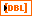signal returns the reconstructed signal.error out contains error information. This output provides standard error out functionality.

## Examples

Refer to the following VIs for examples of using the WA Inverse Discrete Wavelet Transform VI:

• ECG Compression VI: labview\examples\Wavelet Analysis\WAApplications
• Image Compression VI: labview\examples\Wavelet Analysis\WAApplications
• Get and Set Single Level Detail Coefficients VI: labview\examples\Wavelet Analysis\WAGettingStarted
• Wavelet Transform and Reconstruction with Image VI: labview\examples\Wavelet Analysis\WAGettingStarted
• Piece Polynomial Function Approx and Comp VI: labview\examples\Wavelet Analysis\WAGettingStarted
• Get and Set Approximation Coefficients VI: labview\examples\Wavelet Analysis\WAGettingStarted